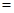# In the reaction of two moles of gaseous hydrogen and one mole of gaseous oxygen to form two moles of gaseous water vapor, two moles of products are formed from three moles of reactants. If this reaction is done at 1.01 × 10 4 Pa (and at 0 °C), the volume is reduced by 22.4 L. (a) In this reaction, how much work is done on the system (H 2 , O 2 , H 2 O) by the surroundings? (b) The enthalpy change for this reaction is −483.6 kJ. Use this value, along with the answer to (a), to calculate Δ r U , the change in internal energy in the system.### Chemistry & Chemical Reactivity

9th Edition
John C. Kotz + 3 others
Publisher: Cengage Learning
ISBN: 9781133949640

#### Solutions

Chapter
Section### Chemistry & Chemical Reactivity

9th Edition
John C. Kotz + 3 others
Publisher: Cengage Learning
ISBN: 9781133949640
Chapter 5, Problem 120SCQ
Textbook Problem
3 views

## In the reaction of two moles of gaseous hydrogen and one mole of gaseous oxygen to form two moles of gaseous water vapor, two moles of products are formed from three moles of reactants. If this reaction is done at 1.01 × 104 Pa (and at 0 °C), the volume is reduced by 22.4 L. (a) In this reaction, how much work is done on the system (H2, O2, H2O) by the surroundings? (b) The enthalpy change for this reaction is −483.6 kJ. Use this value, along with the answer to (a), to calculate ΔrU, the change in internal energy in the system.

(a)

Interpretation Introduction

Interpretation:

The work done on the system by the surroundings has to be determined

Concept Introduction:

The standard enthalpy of formation is the enthalpy change for the formation of 1mol of the compound directly from its component elements in their standard states.

Enthalpy change for the reaction ΔrH0ΣnΔfH0(products)-ΣnΔfH0(reactants)

### Explanation of Solution

Given,

Volume=22.4L

Pressure=1.01×105Pa

Substitute in W =-P(ΔV) as,

W=-(22.4L×1

(b)

Interpretation Introduction

Interpretation:

The change in internal energy in the system has to be calculated.

Concept Introduction:

Standard enthalpy of the reaction,ΔrHo, is the change in enthalpy that happens when matter is transformed by a given chemical reaction, when all reactants and products are in their standard states.

Enthalpy change for the reaction ΔrH0=ΣnΔfH0(products)-ΣnΔfH0(reactants)

### Still sussing out bartleby?

Check out a sample textbook solution.

See a sample solution

#### The Solution to Your Study Problems

Bartleby provides explanations to thousands of textbook problems written by our experts, many with advanced degrees!

Get Started

Find more solutions based on key concepts
A slice of peach pie supplies 357 calories with 48 units of vitamin A; one large peach provides 42 calories and...

Nutrition: Concepts and Controversies - Standalone book (MindTap Course List)

Review. Find every angle between 0 and 360 for which the ratio of sin to cos is 3.00.

Physics for Scientists and Engineers, Technology Update (No access codes included)

Where would you look for a benthic organism?

Oceanography: An Invitation To Marine Science, Loose-leaf Versin